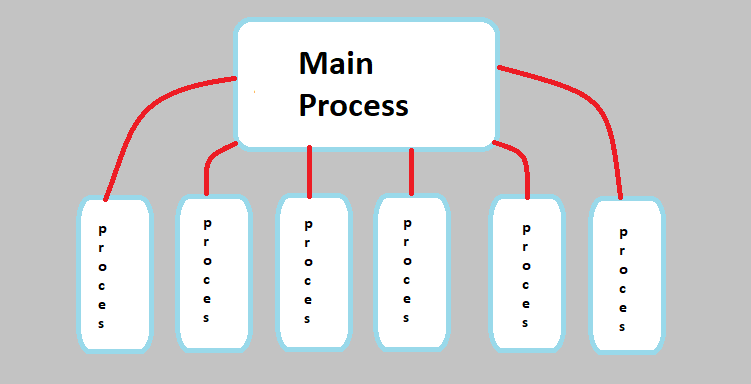09/07/2019 18

## Multi Threading in C# - Working with Multi Channels

Hello everyone, now we are gonna talk about Multi Threading in C#. Firstly I will talk about What Multi Threading is. Multi threading stands for working with multi channel. So there is a mission and our program fulfills this mission with a little processes. With multi process a program can be more powerful and efficient. If a tap fill a pool in an hour, two taps can do this in 30 minutes (if they same types :D ). In this way, our operations will accelerate twice as three times. Because we will be trading with more than one thread. . Firstly we need to import Threading library in our project.
``````
``````
After adding the above libraries, we can go to the coding section. Now we need to specify the methods that will work as thread. It means when thread run specified function will run. We need to prepare this.
``````
public void count1()
{
for (int i = 0; i < 10; i++)
{
Console.WriteLine(i.ToString());
}
}

public void count2()
{
for (int i = 0; i < 10; i++)
{
Console.WriteLine(i.ToString());
}
}

public void count3()
{
for (int i = 0; i < 10; i++)
{
Console.WriteLine(i.ToString());
}
}
``````
We have created three methods and we will call them in three seperate threads. Now take a look at how they work :
``````
treatd.Start();

``````
The code block above we have created Threads and started them. As parameter of these threads we set methods which we created above section. As you can see we have declerated and started these methods bounded by these threads. Result of the program will be similar to below section. This is for my computer, probably result will be different for your computer because of processor working.
``````
1
2
1
2
3
4
1
2
6
7
3
4
5

``````
I did not write here all of them. Now we know how will go on.

## What If we want to call same method in seperate threads ?

``````
void my_method()
{
for (int i = 0; i < 10; i++)
{
Console.WriteLine(i.ToString());
}
}

``````
As you can see above we have called same function with three seperate threads. If this is a function that must have work more more times, we can do this in shorter time with this threads. Result will be same as above section mixed. If we want to call same function with different start and end parameter values we can do this like below example :
``````
void function(int start,int end)
{
for (int i = start; i < end; i++)
{
Console.WriteLine(i.ToString());
}
}

``````
We have started this function twice with different parameter values. The result will be similer to below section :
``````
1
2
10
11
12
3
14
4

``````
Another method is to start threads with delegates. It means we equalize  codes to a delegate and run it in this way. Here is the example of this :
``````
{
for (int i=0; i<10; i++)
{
for (int i = 0; i < 10; i++)
{
Console.WriteLine(i.ToString());
}

}
};
``````
Now let me make a last example and finish the article. Our program will find even numbers until a given number. Our program will do this with multi threads.
``````
int count= 0;

void check_even_number(int start,int end) //control function
{
for (int i = start; i < end; i++)
{
if (i % 2 == 0)
{
count++;
Console.WriteLine(i.ToString());
}
}
}

int thread_count= entered_number / 1000 + 1;

for (int i = 0; i < thread_count; i++)
{
}
``````
We will divide numbers by 1000 to check, entered number will divide by 1000 and create threads to divide this workload. We have used to for loop to start needed quantity threads. As you can see above we have created threads as we need quantity. So our mission divided by multiple process, our program gained power and been more efficient.

Have a nice coding :)

Burak Hamdi Tufan